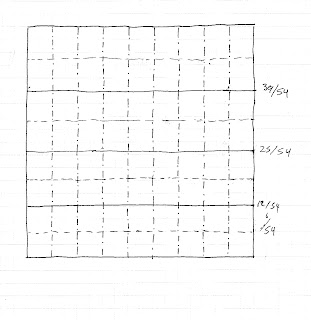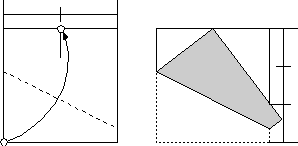## Sunday, May 25, 2008

### The Haga Theorems (Part I)Months ago, when I get envolved with Robert Lang's Miura Ken Rose I got the courage of writing him an email, to show him my humble solution of its CP (now its diagram was published on Origami USA 2007 Convention Book and 12th JOAS Convention) and his answer filled me with happiness, since he not only authorized me to put my doc online, but also gave me several suggestions and good advices.

One of them was a "Junior" version of the Miura Ken, described by him as the construction of a grid with horizontal divisions of 12/54, 25/54, and 39/54, each one of them divided also in half, along with vertical divisions of 1/9ths. I've been faced then with the problem of generate these strange and kind of bizarre proportions of 1/54ths. But obviously is not like we need to fold halfs until reach 1/54, if we consider that 6/54 are 1/9 and that 2 times that makes the first of the suggested divisions . Also, if we get these 6/54ths units, 6.5 times them are the 39/54 for the lowest division, and to fold one of these units in half doesn't sound so terrible.Then, how to create a 1/9th fold? The search for the answer lead me to the Haga Theorems, described perfectly on the Japan Origami Academic Society website, by somebody identified as Koshiro. The idea then is to show how they works and in what they are based.

First Haga Theorem says more or less this: "If we take the corner of a square to a mark created from a odd division on the opposite side, it will show an indication of a known even division on the adyacent side". To visualize this we will take the simplest case on dividing a side in two and get the opposite corner to this mark on the side.As we can see, we obtained an indication of 2/3 on the right side. Its explanation comes fom the world of Geometry (of course). Triangles SAP and PBT are related, it is said that they are "similars" or proportionals, this is: the second one is equal but proportionally greater than the first one; its demonstration is based on their inner angles, there is a classic theorem on geometry (and here I use the word "classic" to avoid me to demonstrate it :D ) which says that if a triangle has every side perpendicular to one of the sides of another triangle, then their inner angles are the same and the triangles are similar or proportionals. In this case, it is obvious that side SA is perpendicullar to PB (a segment of side AB), that side AP is perpendicullar to BT and that SP it is to PT.

Then SA=c*PB, AP=c*BT y SP=c*PT and we know AP=PB=1/2 and that SA+SP=1, we wish to know BT.

If the triangles are proportionals,

AP/SA=BT/PB

AP/SA=BT/(1-AP)
(1/2)/SA=BT/(1/2) and then BT=1/(4*SA)

How much is SA then? Pithagoras says thatand then SA = (1-1/4)/2 = (3/4)/2 = 3/8

and BT = 1/(4*3/8) =1/(3/2) = 2/3

Now, the general case shows thatgenerating the following table, which could be our best friend when creating arbitrary grids is needed:in a future entry we could review the other forms to obtain this table, known as Second and Third Haga Theorems, if there is the interest of course :P

muchos saludos...

#### 1 comment:

Alon said...

I saw you got no comments for this post so…
I teach gifted kids and this year Im going to open a class called Mathematical origami. One of the subjects will be paper division (which will lead to a discussion on real rational and irrational numbers). Haga theorem will be the highlight of the topic (brought as a research project). In spite of the language barrier, they will probably be sent to your post.